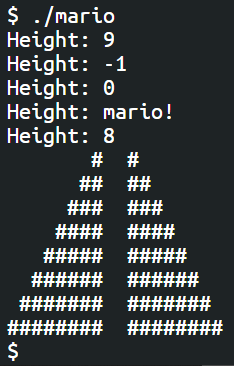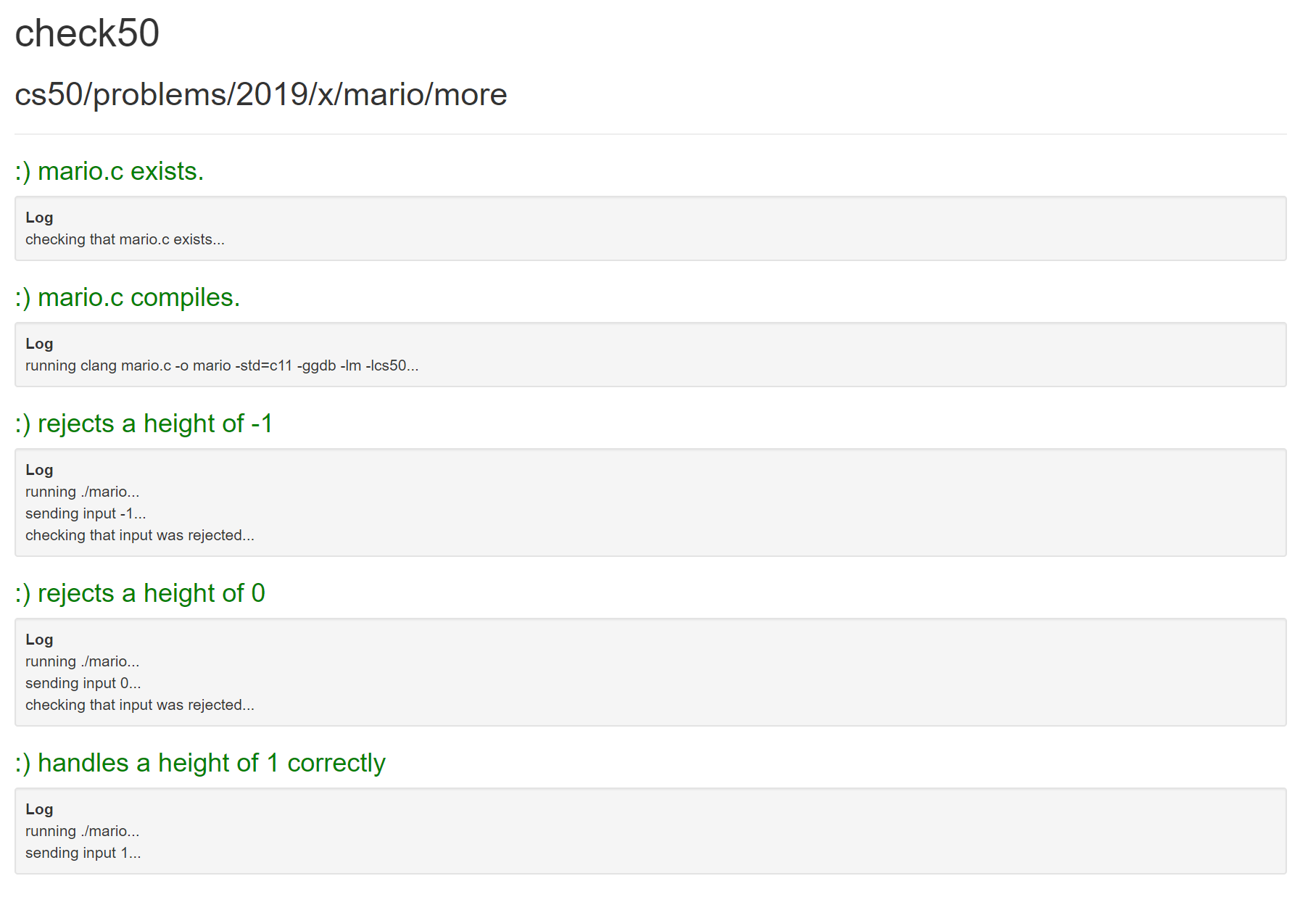# cs50 week 1: review

4 minute read

cs50 is essentially a weekly two-hour lecture followed by what can seem like a million hours of head-scratching, googling, and trial-and-error-ing to solve the problem sets. Week 1 (2 for humans…) was no different, but did result in an epiphany for me in how I can approach my problems in a more methodical and purposeful way. My full solution (a good old-fashioned nested ‘for’ loop in C) is at the bottom of this post.

This took me an age, mostly of bunging numbers in and compiling to see if it worked. My fatal error was jumping in to writing the code without really thinking about what I was doing, so after several hours it struck me that trial and error probably wasn’t the best way to learn!

After a bit of pen and paper thinking I came up with the below approach.

## How I did it (eventually)

I started thinking of the spaces and hashes as empty and filled rows and columns rather than triangle shapes. Plotting them out in Excel really helped:Setting it out like this with `n = 1` and so on helped me see that the spaces and hashes are really just two linear series that increase or decrease up to a maximum set by the user.

I then broke down the program’s required behaviour to the following chunks:

1. Validate user input so that only numbers between 1 and 8 are accepted.

2. Print `<n -1>` spaces, 1 hash, 2 spaces and 1 hash for the first row:

`# #`

3. Repeat, subtracting 1 from the number of spaces and adding 1 to the number of hashes for each row.

4. Stop when the number of rows equals the user’s input.

## Validate user input

First is validate user input. This is relatively simple (it still took me a while), as you can customise the `do while` loop from the `get_positive_int()` function example in the CS50 material.

``````int user_input;
do
{
user_input = get_int("Height: ");
}
while (user_input < 1 || user_input > 8);
``````

This initiates the integer `user_input`, then executes the code inside the curley braces without checking any conditions.

After the code has executed once, it checks that user input wasn’t less than 1 or greater than 8. If it was, it repeats the `do` code.

I initially tried this with `if` and `else` statements but it became too long winded. You could also use a `for` loop, but this would require more lines. As you already know that you want to ask the user for input at least once, the `do` loop made most sense to me here.

## Loop for rows

This is where my mind got tied up in knots. After mapping the output in Excel, I eventually arrived at the conclusion that I needed one loop for the rows and within that another set of loops for the column characters.

Nesting loops like this really emphasised to me the value of giving useful names to variables. Initially I started with calling counter variables `i` and `n`, but when I started using `user_input`, `row`, `column`, `spaces` and `hashes` I found it so much easier to work with.

Here’s the higher level loop for rows:

``````// Row loop
for (int row = 1; row <= user_input; row++)
{
// Column code goes here
}
``````

This just creates a counter variable called `row` and executes the column code until it has reached the number of `user_input`.

## Loop for columns

Within the row loop to create new rows is the column loop to create new columns. These columns have a decreasing number of spaces (from `user_input - 1` to `0`) and an increasing number of hashes (from `1` to `user_input`).

The `for` loops in here refer to the `row` variable from the parent loop.

``````// Column loop - spaces
for (int spaces = user_input - row; spaces > 0; spaces--)
{
printf(" ");
}

// Column loop - left hand hashes
for (int hashes = 1; hashes <= row; hashes++)
{
printf("#");
}

// Spaces between the hashes
printf("  ");

// Column loop - right hand hashes
for (int hashes = 1; hashes <= row; hashes++)
{
printf("#");
}

// New row
printf("\n");
``````

Put it all together and you have a working mario.c:And from check50:## Here’s what I learned

I was surprised at how much I learned from a basic programming problem. The crucial part for me was the decision to stop hacking away with trial and error and instead think logically about what I needed to achieve.

Here’s what I’m taking away from this problem:

• Rather than jumping in and starting with code, I’ll break the problem down in to chunks and use pseudocode to work out what I want to do.
• Basic maths/algebra might help me with this.
• I’ll use descriptive variable names, even for counters.

Thanks for reading!

Tags:

Categories:

Updated: